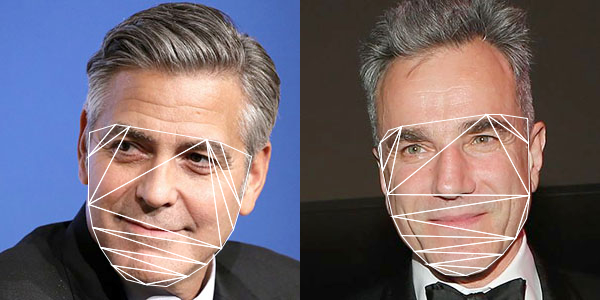Delaunay triangulation of the face contour in OpenCV with Processing

The 4th exercise is a demonstration of the planar subdivision function in OpenCV to retrieve the Delaunay triangulation of the face convex hull outline that we obtain from the last post. The program will use the Subdiv2D class from the Imgproc module in OpenCV.

`Subdiv2D subdiv = new Subdiv2D(r);`

where r is am OpenCV Rect object instance defining the size of the region. It is usually the size of the image we are working on. For every point on the convex hull, we add it to the subdiv object by,

`subdiv.insert(pt);`

where pt is an OpenCV Point object instance. To obtain the Delaunay triangles, we use the following codes,

```MatOfFloat6 triangleList = new MatOfFloat6(); subdiv.getTriangleList(triangleList); float [] triangleArray = triangleList.toArray();```

The function getTriangleList() will compute the Delaunay triangulation based on all the points inserted. It will return the result in the variable, triangleList. This variable is an instance of MatOfFloat6, and which is a collection of 6 numbers. The first pair of numbers are the x and y position of the first vertex of the triangle. The second pair of numbers are for the second vertex. The third pair of numbers are for the third vertex of the triangle. Based on this, we can draw each triangle in the Delaunay triangulation process, as shown in the image below.Complete source code is now available in my GitHub repository at ml20180819b.